# Community

Blog Events Webinars Tutorials Forum
×
Community Blog Straight Talk about "Dynamic Planning"

# Straight Talk about "Dynamic Planning"

By Zhenyu from Cainiao Network

First, let's look at the official definition of dynamic planning:

• Dynamic planning is a branch of operations research, which is the process of seeking the optimization of solution policies. (taken from Baidu Baike)
• The dynamic planning algorithm solves each sub-problem once and stores the results in a table, thus avoiding recalculation of the answers every time each sub-problem is encountered. (taken from Introduction to Algorithms)

## Prerequisites

• Optimization Principle: An optimization policy has such a nature that says regardless of the past state and decision, the remaining decisions must constitute the optimal policy for the state formed by the previous decision. In short, a sub-policy of an optimization policy is always optimal. A problem satisfies the optimization principle, also known as its optimal substructure attributes. (edited by Yuan Pingbo, Gu Weibing, Yin Dong. Data Structures, Applications and Algorithms: University of Science and Technology of China Press, 2013-09)
• No Aftereffect: After each stage is arranged in a specific order, the previous stage state cannot directly affect its future decisions for a given stage state but only the current state. In other words, each state is a complete summary of the past history. This is called no aftereffect.

## My Opinion

A dp array is used to store the intermediate state of the calculation, thus avoiding double calculation. (Overlapping subproblems)

### Basic Concepts

The following example is used to introduce the basic concepts of dynamic planning. Now, there are enough 1 CNY, 2 CNY, and 5 CNY coins, and we need to gather n yuan by finding the minimum number of coins used. Assuming that n is 10099, the mapping analysis is listed below: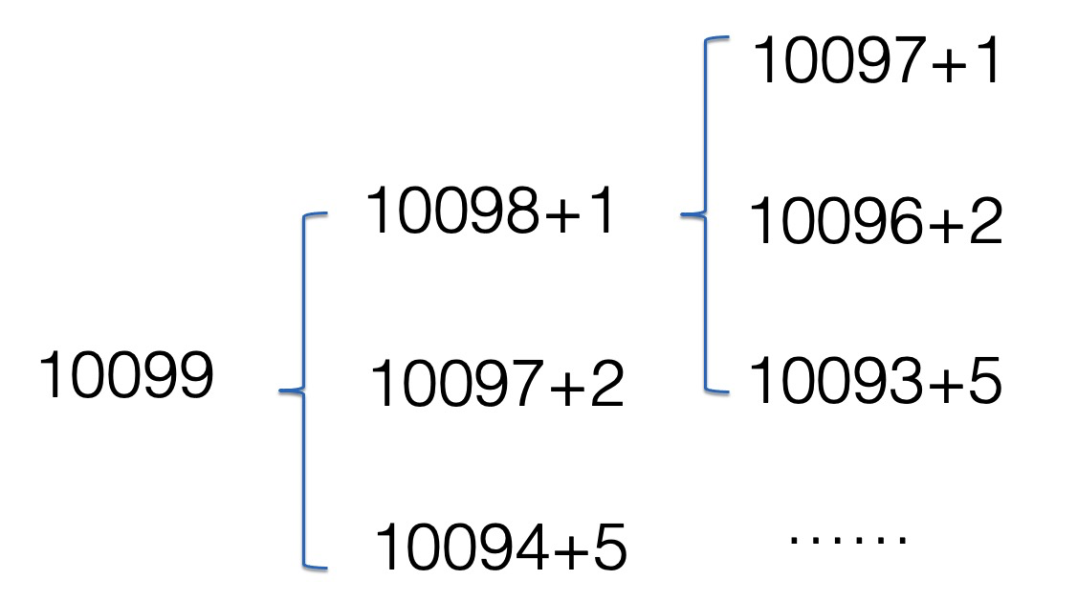The figure shows that dp is calculated twice repeatedly. When the number is smaller, it is calculated more times repeatedly. If you use a memo array to record, you can avoid double calculations, which is the core of dynamic planning algorithms that can improve computational efficiency by trading space for time. Therefore, a one-dimensional array dp can be defined to store the intermediate state of the calculation, where dp(i) represents the least number of coins used to gather i elements. That is the memo.

dp = [f(0), f(1), ...,f(10097),…] where f(o) indicates the initial state. From the figure, it can be obtained that dp = min(dp , dp , dp) +1 can be inferred: f(n) = Math.min(f(n-1]), f(n-2), f(n-5)) +1

The preceding formula is the current state transition equation in dynamic planning. In summary, dynamic planning DP = recursive plus cache.

### Difficulties

The current difficulties of dynamic planning are mainly reflected in the following two aspects:

• How to add cache? -> dimension of the DP array and state?
• How to find recursion? -> The solution of the state transition equation

In particular, there is no fixed formula to solve the state transition equation, it can only be obtained from the analysis of the problem.

## Four Steps of Dynamic Planning

The problems of dynamic planning can be solved in the following four steps:

1. Define Cache dp
2. The Initial State
3. State Transition Equation
4. Get the Results from the dp Cache

## LeetCode Instances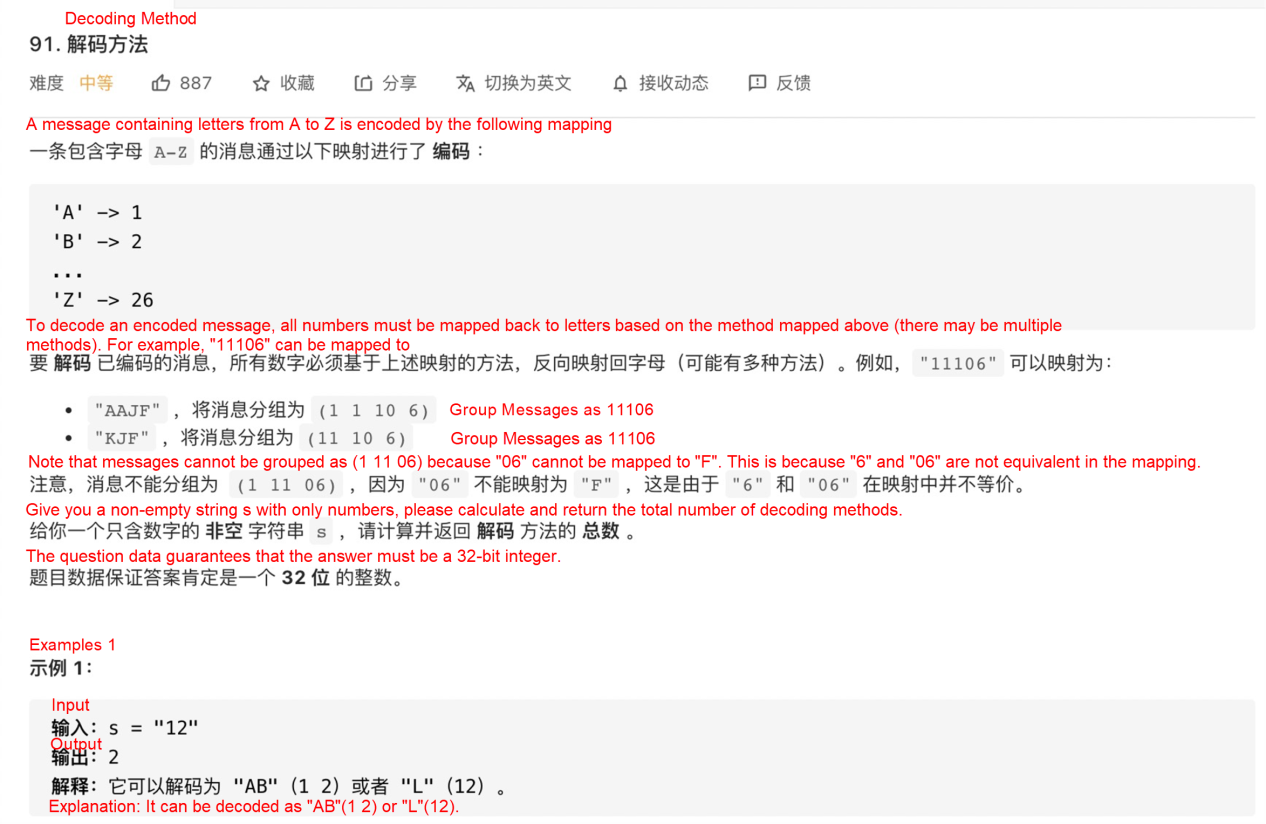### Analysis Process

First, draw and analyze example 1, as shown in the following figure: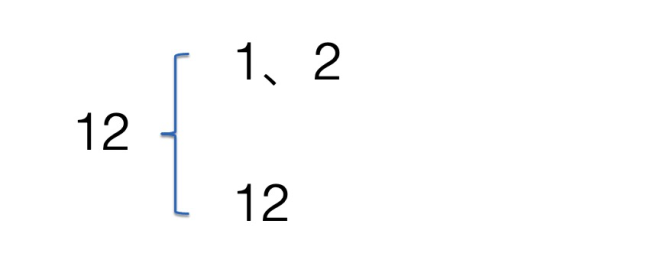After that, consider adding a number X after 12. When X takes 0, it is very special because 0 cannot be transcoded into any letter. It can only be combined with the previous number, as shown in the following figure: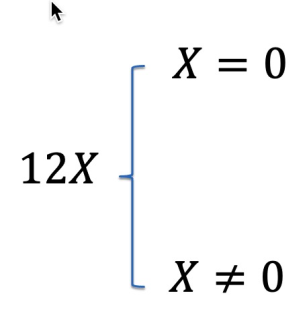At this time, the 2 before X is not representative, so consider converting 2 into the letter P.

When X=0, there are two situations:

• When P is 1 or 2, it can be combined with X=0 to form 10 or 20.
• When P>2, PX cannot be transcoded into letters and returns 0 directly.

When X is not equal to 0, there are also the following two situations:

• If P is equal to 0, X cannot be combined with P. At this time, 1PX and 1P have the same results.
• P is not equal to 0, and it is subdivided into the following two situations:

• If PX > 26, PX cannot be transcoded into letters and can only be split. At this time, the result of 1PX is the same as 1.
• If PX is less than or equal to 26, P and X can be transcoded separately. The result of 1PX is the same as 1P, or PX is transcoded together in combination. In this case, 1PX is the same as the result and 1.

The following figure shows the analysis processes in detail: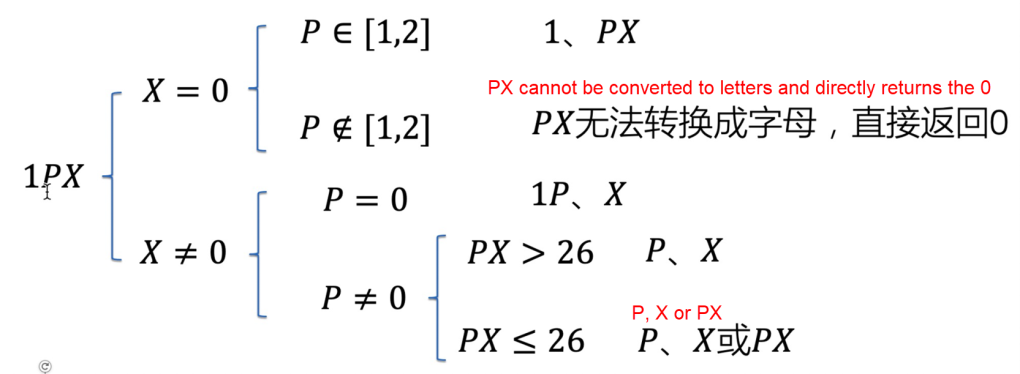### Apply the Four Steps Approach

#### Step 1: Define Cache dp

Define a one-dimensional number dp. The i-th element dp[i] represents the decoded number from the first character to the i-th character.

#### Step 2: Initial State

If the input is not null and the first character is not 0, dp[i]=1. Otherwise, 0 is returned directly.

#### Step 3: State Transition Equation

According to the preceding analysis chart, it is summarized below: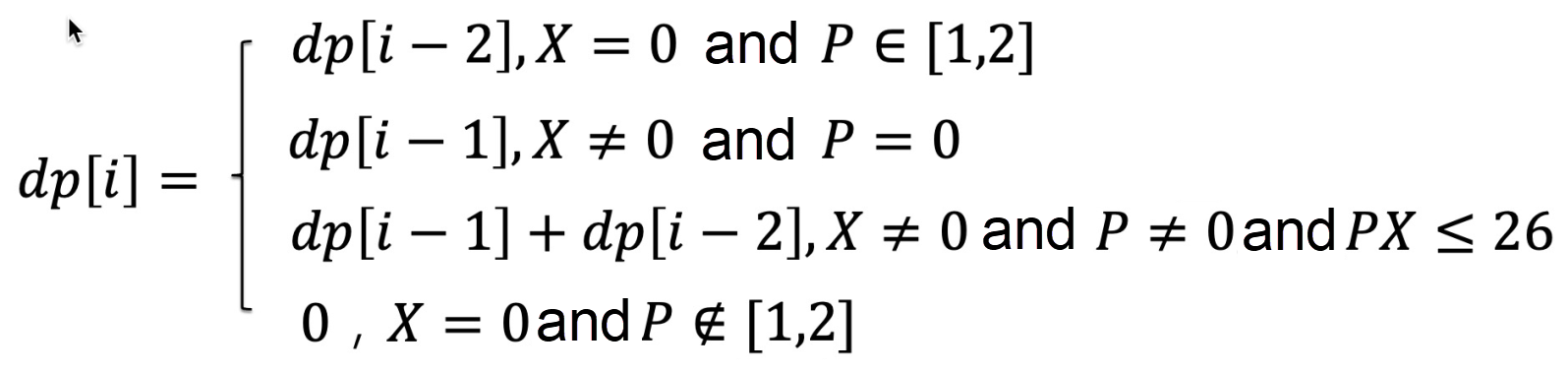#### Step 4: Get the Results from the dp Cache

The last item of the dp array is the solution to the problem.

### Sample Codes

``````var numDecodings = function (s) {
// dp[i] indicates all possible characters of the i-th character
const list = s.split('');
if (list.length === 0 || list === '0') {
return 0;
}
const dp = [];
dp = 1;
for (let i = 1; i < list.length; i++) {
const curChar = list[i];
if (curChar === '0') {
if (['1', '2'].includes(list[i - 1])) {
dp[i] = i >= 2 ? dp[i - 2] : 1;
} else {
return 0;
}
} else {
if (list[i - 1] === '0') {
dp[i] = dp[i - 1];
} else {
const number = Number(list[i - 1]) * 10 + Number(list[i]);
if (number <= 26) {
dp[i] = i >= 2 ? dp[i - 1] + dp[i - 2] : 2;
} else {
dp[i] = dp[i - 1];
}
}
}
}
console.log(dp);
return dp.pop();
}``````

## Summary

Dynamic programming problems still require some experience. It is similar to the process of modeling and requires experience through continuous practice. In summary:

1. Whether there is an association between f(n) and f(n-1),…,f(0)
2. During traversal, a memo can be defined to reduce repeated calculations
3. Remember the four-step solution

Points 1 and 2 are used to determine whether dynamic planning can be used to solve practical problems encountered quickly. Point 3 is a template to keep in mind to solve dynamic programming problems.

0 0 0
Share on